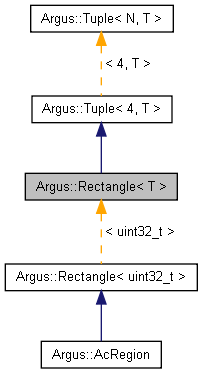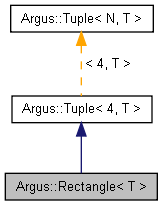## Jetson Linux Multimedia API Reference

#### 32.4.3 Release

Argus::Rectangle< T > Class Template Reference

## Detailed Description

### template<typename T> class Argus::Rectangle< T >

Rectangle template class.

This is a Tuple specialization containing 4 elements corresponding to the positions of the left, top, right, and bottom edges of a rectangle, in that order. Values can be accessed using the named methods.

Definition at line 675 of file Types.h.

Inheritance diagram for Argus::Rectangle< T >:[legend]
Collaboration diagram for Argus::Rectangle< T >:[legend]

## Public Member Functions

Rectangle ()

Rectangle (const Tuple< 4, T > &other)

Rectangle (T init)

Rectangle (T _left, T _top, T _right, T _bottom)

T & left ()

const T & left () const

T & top ()

const T & top () const

T & right ()

const T & right () const

T & bottom ()

const T & bottom () const

width () const
Returns the width of the rectangle. More...

height () const
Returns the height of the rectangle. More...

area () const
Returns the area of the rectangle (width * height). More...

bool operator== (const Tuple< N, T > &rhs) const
Returns true when every element in the two tuples are identical. More...

bool operator!= (const Tuple< N, T > &rhs) const
Returns true if there are any differences between the two tuples. More...

Tuple< N, T > & operator+= (const Tuple< N, T > &rhs)
Adds every element of another tuple to the elements of this tuple. More...

Tuple< N, T > & operator-= (const Tuple< N, T > &rhs)
Subtracts every element of another tuple from the elements of this tuple. More...

Tuple< N, T > & operator*= (const T &rhs)
Multiplies every element in the tuple by a single value. More...

Tuple< N, T > & operator/= (const T &rhs)
Divides every element in the tuple by a single value. More...

const Tuple< N, T > operator+ (const Tuple< N, T > &rhs) const
Returns the result of adding another tuple to this tuple. More...

const Tuple< N, T > operator- (const Tuple< N, T > &rhs) const
Returns the result of subtracting another tuple from this tuple. More...

const Tuple< N, T > operator* (const T &rhs) const
Returns the result of multiplying this tuple by a single value. More...

const Tuple< N, T > operator/ (const T &rhs) const
Returns the result of dividing this tuple by a single value. More...

T & operator[] (unsigned int i)

const T & operator[] (unsigned int i) const

## Static Public Member Functions

static unsigned int tupleSize ()
Returns the number of elements in the tuple. More...

m_data [N]

## Constructor & Destructor Documentation

template<typename T>
 Argus::Rectangle< T >::Rectangle ( )
inline

Definition at line 678 of file Types.h.

template<typename T>
 Argus::Rectangle< T >::Rectangle ( const Tuple< 4, T > & other )
inline

Definition at line 679 of file Types.h.

template<typename T>
 Argus::Rectangle< T >::Rectangle ( T init )
inline

Definition at line 681 of file Types.h.

template<typename T>
 Argus::Rectangle< T >::Rectangle ( T _left, T _top, T _right, T _bottom )
inline

Definition at line 686 of file Types.h.

## Member Function Documentation

template<typename T>
 T Argus::Rectangle< T >::area ( ) const
inline

Returns the area of the rectangle (width * height).

Definition at line 710 of file Types.h.

template<typename T>
 T& Argus::Rectangle< T >::bottom ( )
inline

Definition at line 700 of file Types.h.

template<typename T>
 const T& Argus::Rectangle< T >::bottom ( ) const
inline

Definition at line 701 of file Types.h.

template<typename T>
 T Argus::Rectangle< T >::height ( ) const
inline

Returns the height of the rectangle.

Definition at line 707 of file Types.h.

Referenced by Argus::Rectangle< uint32_t >::area().

template<typename T>
 T& Argus::Rectangle< T >::left ( )
inline

Definition at line 694 of file Types.h.

template<typename T>
 const T& Argus::Rectangle< T >::left ( ) const
inline

Definition at line 695 of file Types.h.

 bool Argus::Tuple< N, T >::operator!= ( const Tuple< N, T > & rhs ) const
inlineinherited

Returns true if there are any differences between the two tuples.

Definition at line 471 of file Types.h.

 const Tuple Argus::Tuple< N, T >::operator* ( const T & rhs ) const
inlineinherited

Returns the result of multiplying this tuple by a single value.

Definition at line 521 of file Types.h.

 Tuple& Argus::Tuple< N, T >::operator*= ( const T & rhs )
inlineinherited

Multiplies every element in the tuple by a single value.

Definition at line 493 of file Types.h.

 const Tuple Argus::Tuple< N, T >::operator+ ( const Tuple< N, T > & rhs ) const
inlineinherited

Returns the result of adding another tuple to this tuple.

Definition at line 509 of file Types.h.

 Tuple& Argus::Tuple< N, T >::operator+= ( const Tuple< N, T > & rhs )
inlineinherited

Adds every element of another tuple to the elements of this tuple.

Definition at line 477 of file Types.h.

 const Tuple Argus::Tuple< N, T >::operator- ( const Tuple< N, T > & rhs ) const
inlineinherited

Returns the result of subtracting another tuple from this tuple.

Definition at line 515 of file Types.h.

 Tuple& Argus::Tuple< N, T >::operator-= ( const Tuple< N, T > & rhs )
inlineinherited

Subtracts every element of another tuple from the elements of this tuple.

Definition at line 485 of file Types.h.

 const Tuple Argus::Tuple< N, T >::operator/ ( const T & rhs ) const
inlineinherited

Returns the result of dividing this tuple by a single value.

Definition at line 527 of file Types.h.

 Tuple& Argus::Tuple< N, T >::operator/= ( const T & rhs )
inlineinherited

Divides every element in the tuple by a single value.

Definition at line 501 of file Types.h.

 bool Argus::Tuple< N, T >::operator== ( const Tuple< N, T > & rhs ) const
inlineinherited

Returns true when every element in the two tuples are identical.

Definition at line 465 of file Types.h.

 T& Argus::Tuple< N, T >::operator[] ( unsigned int i )
inlineinherited

Definition at line 532 of file Types.h.

 const T& Argus::Tuple< N, T >::operator[] ( unsigned int i ) const
inlineinherited

Definition at line 533 of file Types.h.

template<typename T>
 T& Argus::Rectangle< T >::right ( )
inline

Definition at line 698 of file Types.h.

template<typename T>
 const T& Argus::Rectangle< T >::right ( ) const
inline

Definition at line 699 of file Types.h.

template<typename T>
 T& Argus::Rectangle< T >::top ( )
inline

Definition at line 696 of file Types.h.

template<typename T>
 const T& Argus::Rectangle< T >::top ( ) const
inline

Definition at line 697 of file Types.h.

 static unsigned int Argus::Tuple< N, T >::tupleSize ( )
inlinestaticinherited

Returns the number of elements in the tuple.

Definition at line 536 of file Types.h.

template<typename T>
 T Argus::Rectangle< T >::width ( ) const
inline

Returns the width of the rectangle.

Definition at line 704 of file Types.h.

Referenced by Argus::Rectangle< uint32_t >::area().

## Field Documentation

 T Argus::Tuple< N, T >::m_data[N]
protectedinherited

Definition at line 539 of file Types.h.

The documentation for this class was generated from the following file: